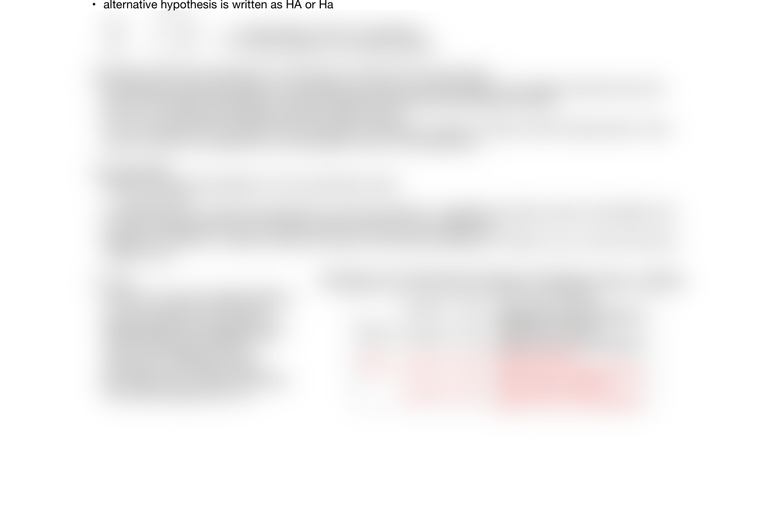Class Notes (1,100,000)
US (480,000)
UCLA (10,000)
STATS (200)
Lecture 4

# STATS 13 Lecture Notes - Lecture 4: Null Hypothesis, Statistical Hypothesis Testing

Department
Statistics
Course Code
STATS 13
Professor
Tsiang, Mike
Lecture
4

This preview shows half of the first page. to view the full 1 pages of the document.Terminology!
Observational units in Buzz and Doris-- particular attempts!
variable? -- whether the button pressed is correct!
categorical !
statistic -- proportion of times buzz pushed corrected number!
parameter !
!
possible vs plausible!
!
Section 1.2 Measuring the Strength of Evidence!
Test of signiﬁcance or hypothesis test!
measure the strength of evidence for or against a chance model!
example 1.2 !
rock-paper scissors!
are these choices used in equal proportions !
study suggests that scissors are chosen 1/3 times less!
two outcome variables = binary variables -- don't necessarily have to be equal!
!
Hypothesis: !
when conducting a test of signiﬁcance, give a null and alternative hypothesis!
null hypothesis = chance explanation!
typically he alternative hypothesis is what the researchers think is true!
ex. null = buzz picks randomly alternative = buzz understands doris!
* these hypothesis are statements about the parameter (long run behavior of the process) not the statistic
(observed result)!
the data itself doesnt need hypothesis because you already have it !
ex. null = people playing rock paper scissors will equally choose between three options. alternative= scissors is
chosen 1/3 less!
null hypothesis is written is Ho, H- naught or H zero!
alternative hypothesis is written as HA or Ha !
!
pi = greek letter not the circumference !
pi = true probability of choosing scissors!
!
signiﬁcance testing is analogous to criminal trial "innocent until proven guilty"!
we assume our chance model (null hypothesis) is true and we collect data and calculate a sample proportion!
the null hypothesis is assumed to be true throughout the signiﬁcance testing procedure!
this is why we use and stimulate using the chance model!
if we conclude that the observed outcome (data) is extremely unusual or unlikely under this assumption, them
(and only then) do we reject the null hypothesis in favor of the alternative!
!
The 3s strategy!
statistic: compute the statistic from the observation data!
p- hat = 1/6!
simulate: identify a model that represents a chance explanation. repeatedly simulate values of the statistic that
could have happened when the chance model is true and form a distribution!
strength of evidence: consider whether the value fo the observed statistic is unlikely to occur when the chance
model is true!
!
P-value!
proportion of the simulated statistics
in the null distribution that are at
least as extreme (in the direction of
the alternative hypotheses) as the
value of the statistic actually
observed in the research study!
the smaller the p-value the stronger
the evidence against the null!
#